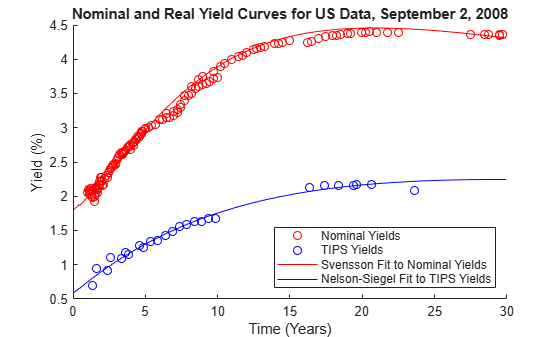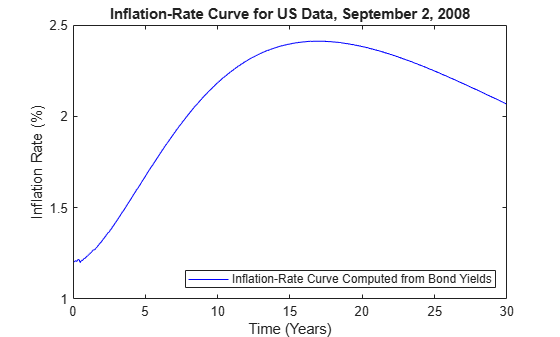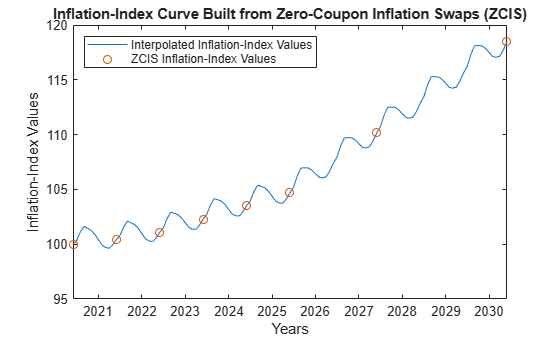Main Content

# Analyze Inflation-Indexed Instruments

This example shows how to analyze inflation-indexed instruments using Financial Toolbox™ and Financial Instruments Toolbox™.

### Compute Real Prices and Yields for Inflation-Indexed Bonds

While inflation-indexed bonds have a great deal of variation in the design, for example, the length of the indexation lag, the majority of inflation-indexed bonds now have a three month lag. They are also capital-indexed, that is, the principal of the bond is indexed to inflation. Therefore, the coupon rate of the bond is constant, but the actual coupon payments vary as the principal of the bond is indexed to inflation.

Specifically, the indexation is done with the following ratio:

`$IndexRatio=\frac{CP{I}_{Ref}}{CP{I}_{Base}}$`

where $CP{I}_{Base}$ is the level of the consumer price index (or equivalent price measure) at the time of the bond's issue and $CP{I}_{Ref}$ is the reference CPI.

Typically, you compute the $CP{I}_{Ref}$ by interpolating between the index data of a known inflation-index curve. To compute the cash flows for an inflation-indexed bond, you simply compute the appropriate reference CPI and Index Ratio.

The market convention for inflation-indexed bonds is to quote the price and yield using the actual (that is, unadjusted) coupon, which means that your quote is a real price and yield. To get a real price and yield, you can use the Financial Toolbox™ functions `bndprice` and `bndyield`. For example:

```Price = 124 + 9/32; Settle = datetime(2009,9,28); Coupon = .03375; Maturity = datetime(2032,4,15); RealYield = bndyield(Price,Coupon,Settle,Maturity); disp(['Real Yield: ', num2str(RealYield*100) '%'])```
```Real Yield: 2.0278% ```

### Construct Nominal, Real, and Inflation Curves

With the advent of the inflation-indexed bond market, real curves can be constructed in a similar fashion to nominal curves. Using the available market data, you can construct the real curve and compare it to the nominal curve.

Note that one issue relates to the indexation lag of the bonds. As stated previously, typically the indexation lag is three months, which means that the inflation compensation is not actually matched up with the maturity or the coupon payments of the bond. While Anderson and Sleath  discuss an approach to resolving this discrepancy, for this example, the lag is simply noted.

You can use the `fitNelsonSiegel` `and` `fitSvensson` functions in the Financial Instruments Toolbox™ to create `parametercurve` objects that fit Nelson-Siegel and Svensson models to real and nominal yield curves in the US. The Nelson-Siegel model typically places restrictions on the model parameters to ensure that the interest rates are always positive. However, real interest rates can be negative, which means that these Nelson-Siegel restrictions are not used in the case below.

```% Load the data. load usbond_02Sep2008 Settle = datetime(2008, 9, 2); NominalTimeToMaturity = yearfrac(Settle,NominalMaturity); TIPSTimeToMaturity = yearfrac(Settle,TIPSMaturity); % Compute the yields. NominalYield = bndyield(NominalPrice,NominalCoupon,Settle,NominalMaturity); TIPSYield = bndyield(TIPSPrice,TIPSCoupon,Settle,TIPSMaturity); % Plot the yields. scatter(NominalTimeToMaturity,NominalYield*100,'r'); hold on; scatter(TIPSTimeToMaturity,TIPSYield*100,'b'); % Fit the real yield curve using fitNelsonSiegel. nInst = numel(TIPSCoupon); TIPSBonds(nInst,1) = fininstrument.FinInstrument; for ii=1:nInst TIPSBonds(ii) = fininstrument("FixedBond",'Maturity',TIPSMaturity(ii),... 'CouponRate',TIPSCoupon(ii)); end TIPSNelsonSiegel = fitNelsonSiegel(Settle,TIPSBonds,TIPSPrice);```
```Local minimum possible. lsqnonlin stopped because the final change in the sum of squares relative to its initial value is less than the value of the function tolerance. ```
```% Fit the nominal yield curve using fitSvensson. nInst = numel(NominalCoupon); NominalBonds(nInst,1) = fininstrument.FinInstrument; for ii=1:nInst NominalBonds(ii) = fininstrument("FixedBond",'Maturity',NominalMaturity(ii),... 'CouponRate',NominalCoupon(ii)); end NominalSvensson = fitSvensson(Settle,NominalBonds,NominalPrice);```
```Solver stopped prematurely. lsqnonlin stopped because it exceeded the function evaluation limit, options.MaxFunctionEvaluations = 6.000000e+02. ```
```% Plot the nominal and real yield curves. PlotDates = (Settle+calmonths(1):calmonths(1):Settle+calyears(30)-1)'; PlotTimeToMaturity = yearfrac(Settle,PlotDates); TIPSNelsonSiegelZeroRates = zerorates(TIPSNelsonSiegel,PlotDates); TIPSNelsonSiegelParYields = zero2pyld(TIPSNelsonSiegelZeroRates,PlotDates,Settle, ... 'InputCompounding', -1, 'OutputCompounding', 2); NominalSvenssonZeroRates = zerorates(NominalSvensson,PlotDates); NominalSvenssonParYields = zero2pyld(NominalSvenssonZeroRates,PlotDates,Settle, ... 'InputCompounding', -1, 'OutputCompounding', 2); plot(PlotTimeToMaturity,NominalSvenssonParYields*100,'r') plot(PlotTimeToMaturity,TIPSNelsonSiegelParYields*100,'b') hold off; title('Nominal and Real Yield Curves for US Data, September 2, 2008') xlabel('Time (Years)') ylabel('Yield (%)') legend({'Nominal yields','TIPS yields','Svensson fit to nominal yields',... 'Nelson-Siegel fit to TIPS yields'},'location','southeast')``````% Create an inflation-rate curve by subtracting the real curve from the % nominal curve. InflationRateCurve = ratecurve("zero", Settle, PlotDates, ... NominalSvenssonZeroRates - TIPSNelsonSiegelZeroRates); figure plot(PlotTimeToMaturity, zero2pyld(... zerorates(InflationRateCurve, PlotDates), PlotDates, Settle, ... 'InputCompounding', -1, 'OutputCompounding', 2)*100,'b'); title('Inflation-Rate Curve for US Data, September 2, 2008') xlabel('Time (Years)') ylabel('Inflation Rate (%)') legend({'Inflation-rate curve computed from bond yields'},'location','southeast')```### Constructing Inflation Curves from Zero-Coupon Inflation Swaps

Inflation-linked derivatives have also experienced growth in the market. Some of the most liquidly traded inflation derivatives are zero coupon inflation swaps (`ZeroCouponInflationSwap`) and year-on-year inflation swaps (`YearYearInflationSwap`).

In a zero-coupon inflation swap, the inflation payer agrees to pay the rate of inflation at maturity (lagged by a certain amount) compounded by the number of years. The inflation receiver typically pays a fixed rate, again compounded by the tenor of the instrument. At the inception of the zero-coupon inflation swap, the fixed rate is set to the projected inflation rate for the life of the swap. This rate is called the "breakeven inflation swap rate" and it is quoted in the market .

Using the notation from Hurd and Relleen, you compute the rate as:

`$\left(1+Rat{e}_{swap}{\right)}^{T}=\left(1+Inflatio{n}_{t-L,t+T-L}{\right)}^{T}$`

where t is the current time, T is the tenor, and L is the lag. 

At maturity, the actual cash flows of the zero-coupon inflation swap are:

`$FixedLeg=N×\left[\left(1+k{\right)}^{M}-1\right]$`

`$InflationLeg=N×\left[\frac{I\left({T}_{M}\right)}{{I}_{0}}-1\right]$`

where

• $N$is the reference notional of the swap.

• $k$ is the fixed inflation rate.

• $M$is the number of years for the life of the swap.

• $I\left({T}_{M}\right)$is the inflation index at the maturity date with some lag (for example, three months).

• ${I}_{0}$ is the inflation index at the start date with some lag (for example, three months).

While the fixed-leg cash flow might be different from the actual inflation-leg cash flow at maturity, the fixed breakeven inflation swap rate of the zero-coupon inflation swap represents the projected inflation rate for the tenor of the swap at inception. You can build an inflation curve from a series of breakeven zero-coupon inflation swap rates starting on the same date and maturing on different dates. Here, the dates are already adjusted with the appropriate indexation lag to simplify the notation:

`$I\left(0,{T}_{1Y}\right)=I\left({T}_{0}\right)\left(1+b\left(0;{T}_{0},{T}_{1Y}\right){\right)}^{{T}_{1Y}-{T}_{0}}$`

`$I\left(0,{T}_{2Y}\right)=I\left({T}_{0}\right)\left(1+b\left(0;{T}_{0},{T}_{2Y}\right){\right)}^{{T}_{2Y}-{T}_{0}}$`

`$I\left(0,{T}_{3Y}\right)=I\left({T}_{0}\right)\left(1+b\left(0;{T}_{0},{T}_{3Y}\right){\right)}^{{T}_{3Y}-{T}_{0}}$`

`$...$`

`$I\left(0,{T}_{i}\right)=I\left({T}_{0}\right)\left(1+b\left(0;{T}_{0},{T}_{i}\right){\right)}^{{T}_{i}-{T}_{0}}$`

where

• $I\left(0,{T}_{i}\right)$ is the breakeven inflation index reference number for maturity date ${T}_{i}$.

• $I\left({T}_{0}\right)$ is the base inflation index value for the starting date ${T}_{0}$.

• $b\left(0;{T}_{0},{T}_{i}\right)$ is the breakeven inflation rate for the zero-coupon inflation swap maturing on ${T}_{i}$.

You can get your inflation curve this by using the `inflationbuild` function to create an `inflationcurve` object. To build an `inflationcurve` from zero-coupon inflation swap rates, first define the base inflation date and the corresponding base inflation-index value.

```% Define the base inflation date and index value for the inflation-index % curve. BaseDate = datetime(2020,6,1); BaseIndexValue = 100;```

Then, define the zero-coupon inflation swap rates and the corresponding maturity dates already adjusted with the appropriate indexation lag.

```% Define the zero-coupon inflation swap rates and maturity dates. ZCISTimes = (calyears([1 2 3 4 5 7 10 20 30]))'; ZCISRates = [0.42 0.54 0.76 0.87 0.92 1.39 1.71 2.01 2.46]'./100```
```ZCISRates = 9×1 0.0042 0.0054 0.0076 0.0087 0.0092 0.0139 0.0171 0.0201 0.0246 ```
`ZCISDates = BaseDate + ZCISTimes`
```ZCISDates = 9x1 datetime 01-Jun-2021 01-Jun-2022 01-Jun-2023 01-Jun-2024 01-Jun-2025 01-Jun-2027 01-Jun-2030 01-Jun-2040 01-Jun-2050 ```

In pricing inflation derivatives and building inflation curves, incorporating seasonality can be a critical factor. The zero-coupon inflation swap rates typically have maturities that increase in whole number of years. As a result, the inflation curve is typcially built from zero-coupon inflation swap rates on an annual basis. However, when computing inflation-index values for monthly periods that are not whole number of years, you can make seasonal adjustments to reflect the seasonal patterns of inflation within the year. These 12 monthly seasonal rates are annualized and they add up to zero to ensure that the cumulative seasonal adjustments are reset to zero every year. In the `inflationbuild` function and the `inflationcurve` object, you define these seasonal rates using the `'Seasonality'` name-value pair argument and they are internally corrected to ensure that they add to zero.

```% Define the 12 monthly seasonal rates. % % Months: % Jan Feb Mar Apr May Jun Jul Aug Sep Oct Nov Dec % 1 2 3 4 5 6 7 8 9 10 11 12 % Seasonal Rates (percent): % -6.34 -3.00 -1.34 3.34 5.34 3.66 8.66 5.66 -2.34 -2.66 -4.66 -6.32 SeasonalRates = [-6.34 -3.00 -1.34 3.34 5.34 3.66 8.66 5.66 -2.34 -2.66 -4.66 -6.32]./100```
```SeasonalRates = 1×12 -0.0634 -0.0300 -0.0134 0.0334 0.0534 0.0366 0.0866 0.0566 -0.0234 -0.0266 -0.0466 -0.0632 ```
```% Build an inflation-index curve from zero-coupon inflation swap rates. myInflationCurve = inflationbuild(BaseDate, BaseIndexValue, ... ZCISDates, ZCISRates, 'Seasonality', SeasonalRates)```
```myInflationCurve = inflationcurve with properties: Basis: 0 Dates: [10x1 datetime] InflationIndexValues: [10x1 double] ForwardInflationRates: [9x1 double] Seasonality: [12x1 double] ```

Once you have created the `inflationcurve` object, compute the inflation-index values for each month using `indexvalues`.

```% Compute the inflation-index values. IndexPlotDates = (BaseDate:calmonths(1):BaseDate+calyears(10))'; IndexPlotValues = indexvalues(myInflationCurve, IndexPlotDates);```

To visualize the seasonal patterns of inflation that occur within each year, plot the computed inflation-index values.

```% Plot the inflation-index curve. figure; plot(IndexPlotDates, IndexPlotValues) hold on; plot(myInflationCurve.Dates(1:8), myInflationCurve.InflationIndexValues(1:8), 'o') hold off; title('Inflation-Index Curve Built from Zero-Coupon Inflation Swaps (ZCIS)') xlabel('Years') ylabel('Inflation-Index Values') legend({'Interpolated inflation-index values','ZCIS inflation-index values'},'location','northwest')```### Price Inflation-Indexed Instruments Using an Inflation Curve

With the `inflationcurve` object created, you can price inflation-indexed instruments such as zero-coupon inflation swaps (`ZeroCouponInflationSwap`), year-on-year inflation swaps (`YearYearInflationSwap`), and inflation-indexed bonds (`InflationBond`).

First, create a `ratecurve` object using `ratecurve``.`

```Settle = datetime(2020,9,25); Type = "zero"; ZeroTimes = [calmonths(6) calyears([1 2 3 4 5 7 10 20 30])]'; ZeroRates = [0.0043 0.0051 0.0062 0.0072 0.0096 0.0121 0.0172 0.0241 0.0302 0.0308]'; ZeroDates = Settle + ZeroTimes; ZeroCurve = ratecurve('zero',Settle,ZeroDates,ZeroRates)```
```ZeroCurve = ratecurve with properties: Type: "zero" Compounding: -1 Basis: 0 Dates: [10x1 datetime] Rates: [10x1 double] Settle: 25-Sep-2020 InterpMethod: "linear" ShortExtrapMethod: "next" LongExtrapMethod: "previous" ```

Using the `ratecurve` and `inflationcurve` objects as inputs, create an `Inflation` pricer object using `finpricer`.

`outPricer = finpricer("Inflation",'DiscountCurve',ZeroCurve,'InflationCurve',myInflationCurve)`
```outPricer = Inflation with properties: DiscountCurve: [1x1 ratecurve] InflationCurve: [1x1 inflationcurve] ```

Create an `InflationBond` instrument using `fininstrument`.

```IssueDate = datetime(2020,9,20); Maturity = datetime(2025,9,20); CouponRate = 0.023; InflationBond = fininstrument("InflationBond",'IssueDate',IssueDate,'Maturity',Maturity,'CouponRate',CouponRate) ```
```InflationBond = InflationBond with properties: CouponRate: 0.0230 Period: 2 Basis: 0 Principal: 100 DaycountAdjustedCashFlow: 0 Lag: 3 BusinessDayConvention: "actual" Holidays: NaT EndMonthRule: 1 IssueDate: 20-Sep-2020 FirstCouponDate: NaT LastCouponDate: NaT Maturity: 20-Sep-2025 Name: "" ```

Here, the default indexation lag is three months and the bond issue date is `20-Sep-2020`. The first date on the inflation curve of the pricer must be on or before `20-Jun-2020` to price this instrument. In this example, the first date on the inflation curve of the pricer is `01-Jun-2020`.

Price the `InflationBond` instrument by using the `price` function for the `Inflation` pricer.

`InflationBondPrice = price(outPricer, InflationBond)`
```InflationBondPrice = 110.1314 ```

### References

This example is based on the following papers and journal articles:

 Anderson N. and J. Sleath. "New Estimates of the UK Real and Nominal Yield Curves." Bank of England, working paper 126, 2001.

 Brigo, D. and F. Mercurio. Interest Rate Models - Theory and Practice: With Smile, Inflation and Credit. Springer, 2006.

 Deacon, M., A. Derry, and D. Mirfendereski. Inflation-Indexed Securities: Bonds, Swaps, and Other Derivatives. Wiley Finance, 2004.

 Gurkaynak, R. S., B.P. Sack, and J.H. Wright. "The TIPS Yield Curve and Inflation Compensation." FEDS Working Paper No. 2008-05, October 2008.

 Hurd, M. and J. Relleen. "New Information from Inflation Swaps and Index-linked Bonds." Quarterly Bulletin, Spring 2006.

 Kerkhof, J. "Inflation Derivatives Explained." Lehman Brothers, 2005.

Download ebook